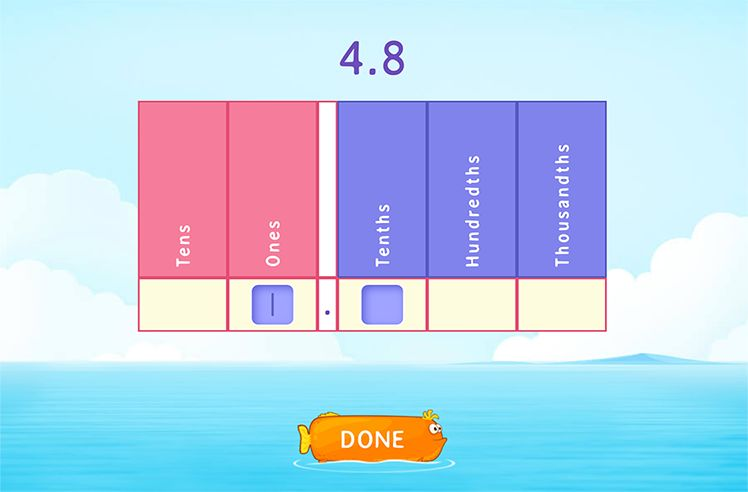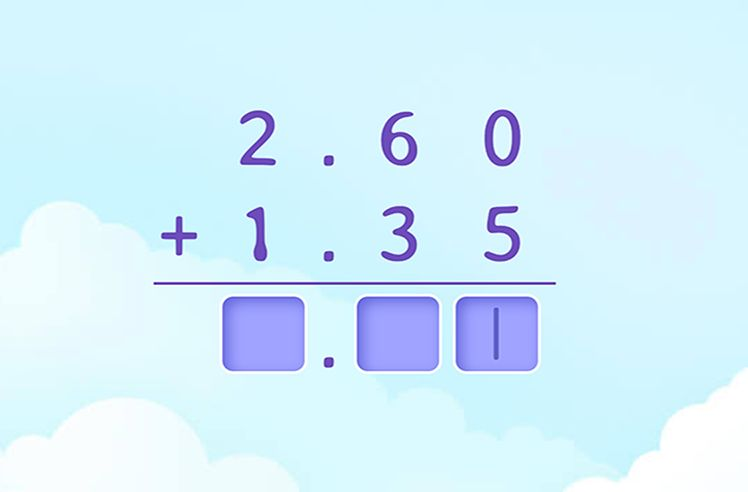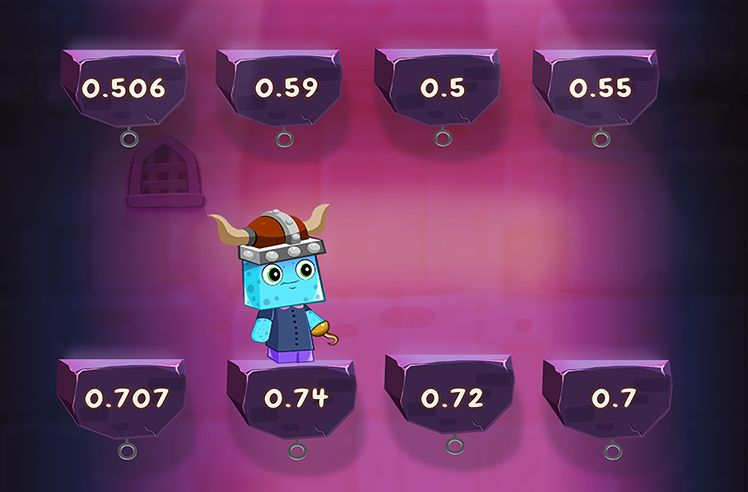# Rounding Decimals – Definition with Examples

» Rounding Decimals – Definition with Examples

## Rounding Decimals

Rounding is a process to estimate a particular number in a context. To round a number look at the next digit in the right place, if the digit is less than 5, round down and if the digit is 5 or more than 5, round up.

Rounding decimals refer to the rounding of decimal numbers to a certain degree of accuracy. We can round decimals to the nearest wholes, tenths or hundredths.

Rounding decimals is useful to estimate an answer easily and quickly. It is also useful to find out the estimate of the average score of the students in a class.

## 1. Rounding to the Nearest Whole

We follow the given steps to round numbers to the nearest whole number:

Step 1- We look at the number we want to round.

Step 2- As we are rounding our number to the nearest whole, we mark the digit in the one’s place.

Step 3- Now we look at the ‘tenths’ place (the digit to the right of the decimal point).

Step 4- (i) If the digit in the tenths column is 0, 1, 2, 3 or 4, we will round down the number at the ones place to the nearest whole number.

(ii) If the digit in the tenths column is 5, 6, 7, 8 or 9, we will round up the number at the one’s place to the nearest whole number.

Step 5- Remove all the digits after the decimal point. The left out number is the desired answer.

Example 1: Round 945.65 to the nearest whole number.

## 2. Rounding to the Nearest Tenths

We follow the given steps to round numbers to the nearest tenths:

Step 1- We look at the number we want to round.

Step 2- As we are rounding our number to the nearest tenths, we mark the digit in the tenths place.

Step 3- Now we look at the ‘hundredths’ place (the digit to the right of the tenths column).

Step 4- (i) If the digit in the hundredths place is 0, 1, 2, 3 or 4, we will round down the number at the tenths place to the nearest tenths.

(ii) If the digit in the hundredths place is 5, 6, 7, 8 or 9, we will round up the number at the tenths place to the nearest tenths.

Step 5- Remove all the digits to the right of the tenths column. The left out number is the answer.

Example 2: Round 542.33 to the nearest tenths.

Example 3: Ryan weighs 27.51 kg. What is his weight to the nearest kg?

The weight to the nearest kg = 28 kg.

## 3. Rounding to the Nearest Hundredths

We can follow the given steps to round numbers to the nearest hundredths:

Step 1- We look at the number we want to round.

Step 2- As we are rounding our number to the nearest hundredths, we mark the digit in the hundredths place.

Step 3- Now we look at the ‘thousandths’ place (the digit to the right of the hundredths column).

Step 4- (i) If the digit in the thousandths place is 0, 1, 2, 3 or 4, we will round down the hundredths place to the nearest hundredths.

(ii) If the digit in the thousandths column is 5, 6, 7, 8 or 9, we will round up the hundredths place to the nearest hundredths.

Step 5- Remove all the digits to the right of the hundredths place. The left out number is the answer.

Example 4: The depth of lake Tanganyika is 1,470.158 m. What is the depth of the lake to the nearest hundredths?Understanding Decimals

Play NowDecimal Operations

Play NowComparing & Ordering Decimals

Play Now